## Homework Assignments—Fall 2001

#### Assignment 1—due 14 September, 2001.

1. Write a program to calculate 1/a, a>0, without using the division instruction,

• using lookup table for first guess

• calculating first guess

• Extra credit: How might you use machine code for a first guess?

2. Write a program to calculate cube roots by Newton's method.

3. Write a set of functions that perform complex arithmetic using only real numbers.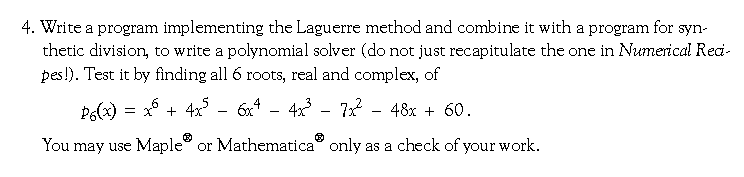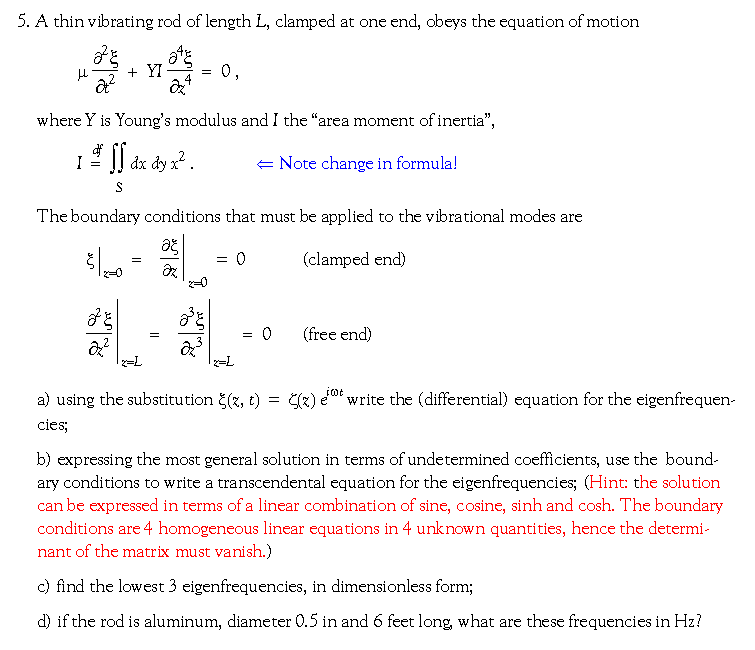(My) Solutions to HW #1

#### Assignment 2—due 01 October, 2001.

1. The “golden section” is defined as the ratio of the sides A and B of the rectangle shown below, such that if a square of side A is cut along the red line, the remaining rectangle will have sides in the same ratio. That is,

A/B = (BA)/A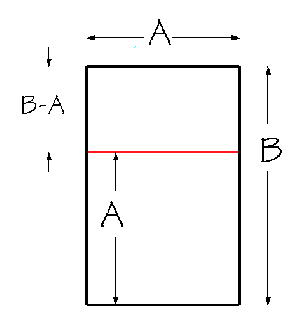What is the ratio A/B for which this is true?

2. Write a program to implement the golden section algorithm for finding function minima. Use it to find minima for the following functions:
• f(x) = –xex×sinh2(x)/[1+cosh2(x)]

• f(x) = x4+x3+8x+8

3. Write a program to find minima by quadratic search. Use it to find the minima of the two functions in the preceding exercise.

4. Write a program to find minima by simulated annealing. Use it to find the minima of the two functions in exercise 2, and also the 2-dimensional function

f(x,y) = r–3 – r–1+r+x

where

r2=x2+y2 .

#### Assignment 3—due 22 October, 2001.

1. The 0'th order Bessel function can be computed by adding terms of the power series given as formula 9.1.10 in Abramowitz & Stegun (p. 360).
1. Write a program to compute the series efficiently by directly adding the series, in the range 0 Ł x Ł 3. The result should have absolute precision of 10–8.
2. Use Chebyshev approximation to find a polynomial fit on the same range that provides the same absolute precision. (Hint: use the table 22.3 found on p. 795 of A&S.)

2. The subroutine below is intended to solve linear equations with tri-diagonal matrices. It has neither comments nor documentation. Without looking at the “Numerical Recipes” book, analyze the subroutine and provide
1. a flow chart;
2. appropriate comments and documentation in the program;
3. a working and tested version in your own favorite programming language (different from the above).
```SUBROUTINE TRIDAG(A,B,C,R,U,N)
PARAMETER (NMAX=100)
DIMENSION GAM(NMAX),A(N),B(N),C(N),R(N),U(N)
IF(B(1).EQ.0.)PAUSE
BET=B(1)
U(1)=R(1)/BET
DO 11 J=2,N
GAM(J)=C(J-1)/BET
BET=B(J)-A(J)*GAM(J)
IF(BET.EQ.0.)PAUSE
U(J)=(R(J)-A(J)*U(J-1))/BET
11    CONTINUE
DO 12 J=N-1,1,-1
U(J)=U(J)-GAM(J+1)*U(J+1)
12    CONTINUE
RETURN
END
```

3. Write a brief program to solve linear systems of equations using pivotal elimination with partial pivoting (that is, the sequence TRIANGULARIZE ® BACKSOLVE ). Use it to solve the test case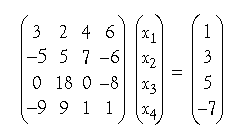to 6 significant figures of precision. If you wish you may use Maple® or Mathematica® or Matlab® to check your work—or else multiply the matrix by the solution and see whether you obtain the inhomogeneous term.

4. Write a short, efficient program to perform bit-reversal of numbers from 0 to 2k – 1 where k=5, 6, ... , 10.
Make sure you also create a tool to test your answer by displaying the input and output in binary format, in a field of k digits, including the leading 0's. (That is, if the standard integer is 32 bits, there should be 32–k leading 0's.)

(My) Solutions to HW #3 (in *.pdf format)

####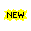Assignment 4—due 26 November, 2001.1. Estimate the time needed to solve the Nth order system of linear equations Ax  =  r using Strassen's “divide and conquer” algorithm. That is, what is the coefficient K in the term KmNlg(7)? (Assume the matrix fits into fast memory, and that there are no delays due to cache misses, etc.)

2. Solve the recursion relation tN = Nl + 2tN/2.

3. Using the inverse formula, a good uniform prng and a root-finder of your choice, generate a table of 10,000 random variates from the semi-circular distribution p(x)=6x(1-x), 0<x<1. Make a histogram of these values and compare with the distribution function.

4. Repeat problem #3 but use the Von Neumann-Metropolis selection method to generate the random variates.

5. Use 5 point Gauss-Hermite integration (look up the points and weights in Abramowitz & Stegun) to evaluate the following integrals on the interval from –¥ to +¥:
1. ex²cosx
2. ex²cos2x
3. ex²cos3x
4. ex²(x4–2x2+1)

Compare the results with the exact answers and discuss the loss of precision (if any!); if you do not know how to do these integrals look them up in tables.

6. Use 5-point Gauss-Laguerre integration to evaluate the integrals (on the interval 0 to +¥)
1. excosx
2. excos2x
3. exsinx
4. ex(x10–2x5+1)

Evaluate the integrals exactly and compare with the numerical results; discuss the loss of precision (if any!) for each case.

My solutions to HW #4 in *.ps format   (in *.pdf format)